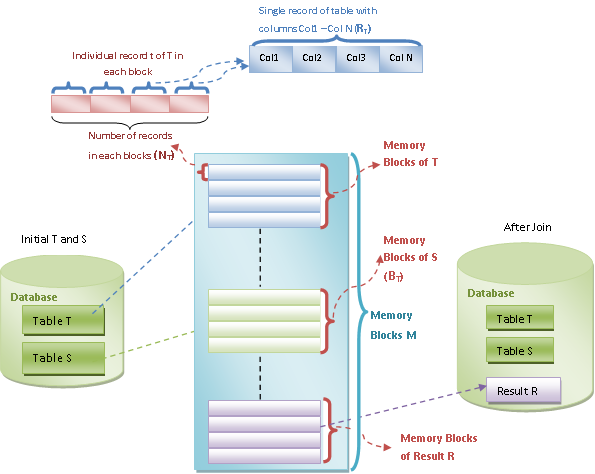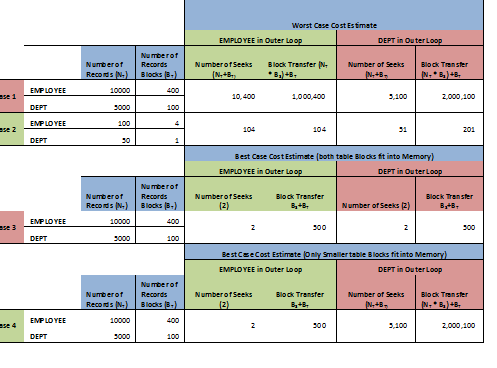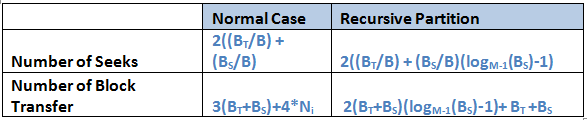# Joins in DBMS

We have different types of join methods defined. When we use the joins in the queries, the query optimizer decides which joins to be used. Based on the better cost of the query, any one of the following join method will be used by the query optimizer.

Suppose we join two tables T and S with joining condition θ. Let t and s be the records in T and S respectively. Suppose T table has BT records in each block and total NT records. Similarly S table has BS records in each block and total NS records. Please have a detailed look at below diagram to understand the difference between different notations used in rest of the article. Please proceed to the topics only when the differences are very clear.## Nested Loop Join (NLJ)

In this method, when we join the tables, inner and outer loops based on the table records are created. The condition is tested in the inner most loop and if it satisfies, then it will be stored in the result set, else next record will be verified. The algorithm for this method can be written as below

```For each record t in T
Loop
For each record s in S
Loop
Check θ on (t, s)
If matches then copy to result set
Else move to next record
End If
End Loop
End Loop```

In above algorithm, we can see that each record of the outer table T is verified with the each record s of the inner table S. Hence it is very costly type of join.
In the worst case, it requires NT +BT seeks and (NT * BS) +BT Block transfers. It is always better to put smaller tables in the inner loop, if it accommodated into its memory. Then the cost will reduce to 2 seeks and BT + BS Block transfers.

Suppose we have EMPLOYEE and DEPT tables with following number of records and blocks. Let us see their costs based on these numbers and the position of the larger and smaller tables. Observe case 1 and 2, where when smaller table DEPT is set as outer table, then the number of block transfer doubles where as number of seek reduces. In the case 3 where both the tables fit into the memory block, the number of seek is reduced to 2 and block transfer also considerably reduces. But we can observe the real difference when only smaller table DEPT fits into the memory and is considered as inner table in case 4. The thumb rule for using tables in outer loop is always smaller table. But when smaller table fits into the memory, then use it in inner loop.ssFrom above examples, we can understand that cost of nested loop join is the game of number of records, blocks and positioning of the tables. However, the cost of nested loop join is very expensive compared to other joins below.

## Block Nested Loop Join (BNLJ)

In this method, in addition to looping each record in outer and inner tables, their blocks are also added for looping. Hence, this method selects block of records from both the tables and compares the records in them. Hence the processing is done block wise.

```For each Block BT in T
Loop
For each Block BS in S
Loop
For each record t in BT
Loop
For each record s in BS
Loop
Check θ on (t, s)
If matches then copy to result set
Else move to next record
End If
End Loop
End Loop
End Loop
End Loop```

Here, it traverses each block of records in a loop. We can observe that, each of outer block reads inner table records only once.  Where as in nested loop join each record in the outer table used to read inner table records. Hence cost of this method has much better worst case cost compared to nested loop method. The number of seeks required is 2 BT and the number of block transfer is given as (BT * BS) + BT. In the best case where records of both table fit into memory block, the cost is given as 2 seeks and BS+ BT block transfers

We can have slight modification in above method while allocating the memory blocks to inner and outer tables. Suppose M is the total available blocks in the memory, BT and BS are the number of records in each block in respective tables. Then allocate M-2 blocks for the outer relation and reaming 2 blocks for the inner and outer mappings. Then the cost will change to 2 (BT / (M-2)) and the number of block transfer is given as ((BT / (M-2)) * BS+ BT). Refer the diagram above if confused.

Suppose the join condition is on candidate key of the tables, then it need not traverse through all the records or blocks of inner table. At one point seek itself, it will get the record.

## Indexed Nested Loop Join (INLJ)

The last sentence of BNLJ above makes us to think what will happen if we have index on the columns used in join condition. When indexes are used, there is no sequential scan of records. That applies here too. When indexes are used on the columns that are used in join condition, it will not scan each records of inner table. It will directly fetch matching record. But we will have the cost for fetching the index in the index table. Indexes are useful when natural joins are joins or equijoins are used. Hence if indexes are not defined on the columns, we can create temporary indexes to run the query.

In the worst case, the cost of INLJ is calculated as BT*(RT+RS) +NT*C, where RT and RS are each records of table T and S respectively, and C is the cost of fetching single record in the index table.

Suppose we have EMP and DEPT tables and index is created on DEPT_ID of EMP. Suppose DEPT is outer table and EMP is inner table. i.e.; DEPT∞DEPT_ID EMP. Suppose B+ tree index of height 4 is used on EMP table and each child node will have 20 indexes fields.

### Assume the value for other variables as below:

• Number of Records in DEPT (NT): 5000
• Number of Records in EMP (NS): 10000
• Number of records per block in T (BT): 100
• Number of records per block in S (BS): 400

Now the cost of fetching single record using index table, C = Height of B+ tree to fetch the index (h) + One seek to retrieve the actual record from the memory = 4 +1 = 5
RT+RS can be ignored here as we are fetching only one record.

Let see the total cost of each type of join below (block transfers and seeks):
Cost of NLJP = (NT+BT) + (NT * BS) +BT = (5000+100) + (5000*400) +100 = 20, 05,200
Cost of BNLJ = 2 BT + (BT * BS) + BT = 2 * 100 + (100*400) + 100 = 40,300
Total cost of the INLJ = BT*(RT+RS) +NT*C =100 + (5000 * 5) = 25,100

From above example we can clearly make out the difference among all the three types of joins. INLJ is the most efficient one among all.

## Merge Join

Tables used in the join query may be sorted or not sorted. Sorted tables give efficient costs while joining. In this method, the column used to join both the tables is used to sort the two tables. It uses merge-sort technique to sort the records in two tables. Once the sorting is done then join condition is applied to get the result. Joining is also similar to any other joins, but if two records have same column value, then care should be taken to sort records based on all the columns of the record. This method is usually used in natural joins or equijoins. Assume that all the records can be accommodated into the memory block. The each block of records is read only once to join. Then the cost of this join would be

Cost of Seeks: BT/M + BS/M
Cost of Block Transfer: BT + BS
If tables are not sorted, then cost for merge sorting is also included in above cost.

## Hash Join

This method is also useful in case of natural and equijoins. We use hash function h on the joining column, to divide the records of each tables into different blocks. Assume each of these hash divided block of records fit the memory block and we have NH number of hash divided blocks.

DT0, DT1, DT2, DTN : are the hash divided blocks of table T, where DTi = h (RT (joinCol)) and RT is in any one of the record in table T and is in partition DTi
DS0, DS1, DS2, DSN : are the hash divided blocks of table S, where DSi = h (RS (joinCol)) and RT is in any one of the record in table S and is in partition DSi

When the tables are partitioned, one memory block is reserved for the input and one memory block is reserved for the output buffer of each partition. Rest of the memory blocks are allocated for the input buffers and outputs equally. Then the hash algorithm can be written as below

```For each partition i Loop
Load DSi into memory and build a hash index on the joining column
Retrieve each record DTi from memory, for each record in DSi build a hash index on joining column and find the matching record in DSi
If matching records are found, then join the records RT and RS into output
End loop
End Loop```

For example, take EMP and DEPT tables, with joining column as DEPT_ID. A hash function on DEPT_ID is used to generate the hash divided block (hash partition) for each of the table. Suppose it generates 5 partitions for each table. According to the algorithm,

### If total number of memory block is M, and the number of hash partition is i, then

• i<M: Above hash algorithm is used to join the tables.
• i>=M: Recursive partitioning method is used to join the tables. That is, both tables are partitioned into M-1 blocks, and proceed with joining and repeat the partition again for rest of the blocks.

There are some cases where some bad hash function or because of join condition, we will get multiple same records. In such case, it is difficult to accommodate all the records into one partition block. This is called hah table overflow. This can be avoided by carefully partitioning the tables. This can be resolved while creating the partition itself by creating sub partitions using another hash function. But both the tables should be partitioned in same way. But we will still have overflow, if tables have lot many duplicate values and in such case we have to use BNLJ method.

There is another issue called skewed partition where some partition will have relatively large number of records compared to other partitions. This is also not good for joining the tables.

Let us see the cost of hash joins.Let us consider an example. Assume
Total number of blocks in memory, M = 20
Number of blocks in EMP, BT = 100
Number of blocks in DEPT, BS= 400
Total number of Partition, I = 5

Then, each partition of EMP will have 20 blocks each and DEPT will have 80 each. That means in the case of EMP i=M.

Hence, total Seeks = 2((BT/B) + (BS/B) = 2((100/3) + (400/3)) = 336
Total block transfer = 3(BT+BS) +4*Ni = 3(100 + 400) = 1500, where 4Ni is ignored because we did not consider partially filled partitions.

Translate »